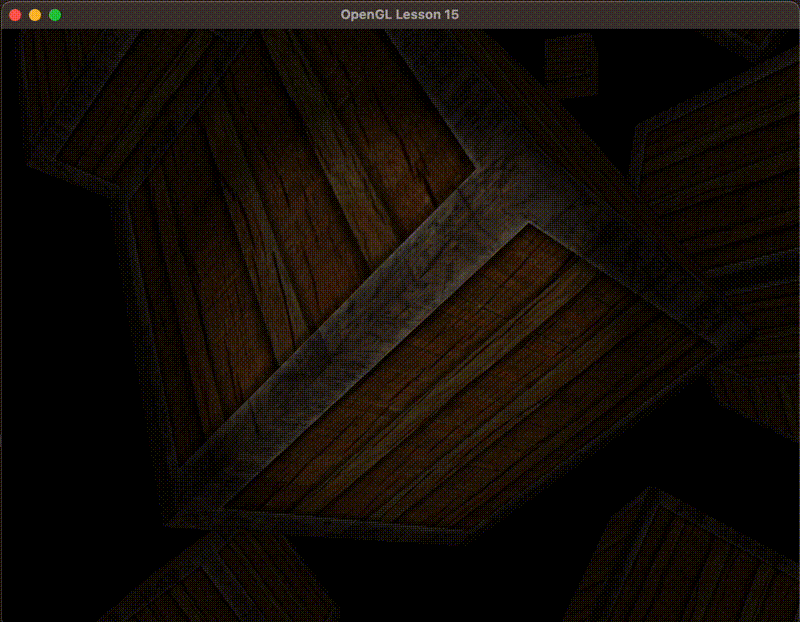# OpenGL2.3投光Caster

2023-01-27

## 平行光struct Light {
vec3 direction;

vec3 ambient;
vec3 diffuse;
vec3 specular;
};

...

void main()
{
vec3 lightDir = normalize(-light.direction);
...
}


vec4正是旧OpenGL（固定函数式）决定光源是定向光还是位置光源(Positional Light Source)的方法，并根据它来调整光照。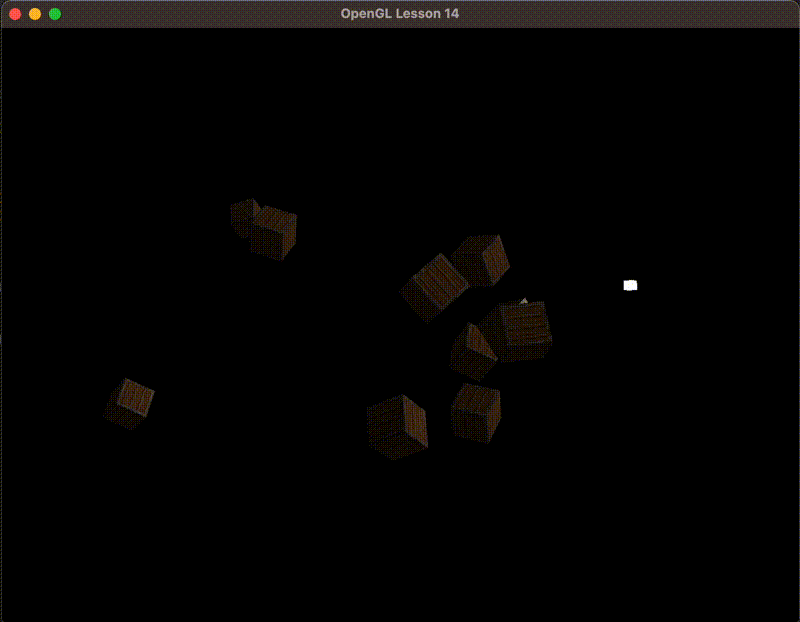## 点光源### 衰减函数

$F_{att} = \frac{1.0}{K_c + K_1 * d + K_q * d^2}$

1. $K_c$通常为1, 主要是为了确保分母始终大于1, 不至于是强度超过1.0
2. 一次项常数$K_1$与距离相乘，以此来按照距离线性减少光的强度。
3. 二次项常数$K_q$与距离的平方相乘，按照平方减少的特点是，距离短的时候影响不大，距离大的时候就超过一次项的影响了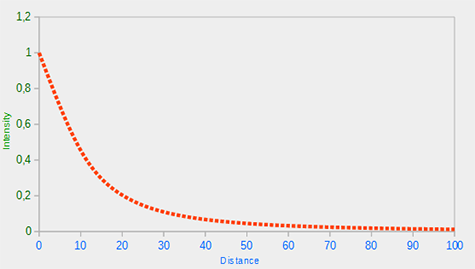### 数值表

Distance(d) Constant($K_c$)   Linear($K_1$)   Quadratic($K_q$)
7 1.0 0.7 1.8
13 1.0 0.35 0.44
20 1.0 0.22 0.20
32 1.0 0.14 0.07
50 1.0 0.09 0.032
65 1.0 0.07 0.017
100 1.0 0.045 0.0075
160 1.0 0.027 0.0028
200 1.0 0.022 0.0019
325 1.0 0.014 0.0007
600 1.0 0.007 0.0002
3250 1.0 0.0014 0.000007

struct Light {
vec3 position;

vec3 ambient;
vec3 diffuse;
vec3 specular;

float constant;
float linear;
};

float distance = length(light.position - FragPos);
float attenuation = 1.0 / (light.constant + light.linear * distance + light.quadratic * (distance * distance));


glUniform1f(glGetUniformLocation(shaderProgram, "light.constant"),  1.0f);


ambient  *= attenuation;
diffuse  *= attenuation;
specular *= attenuation;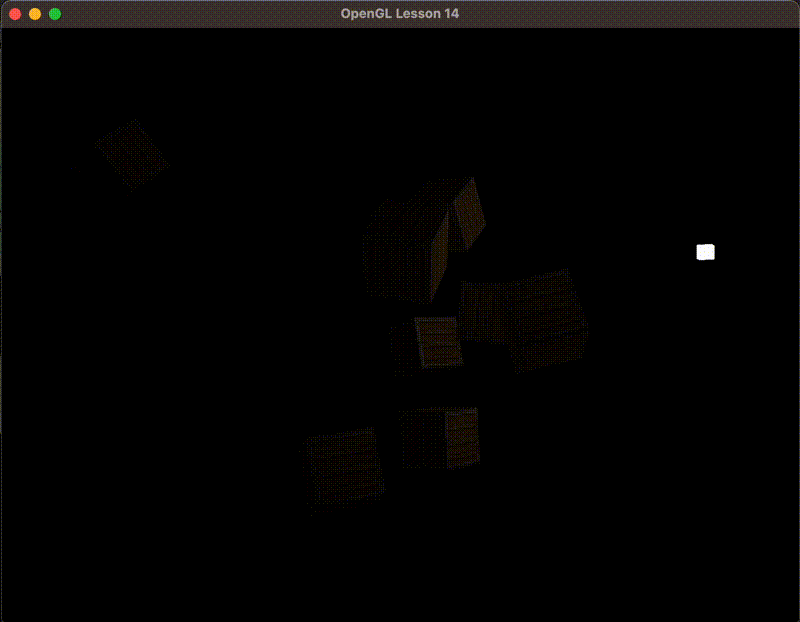## 聚光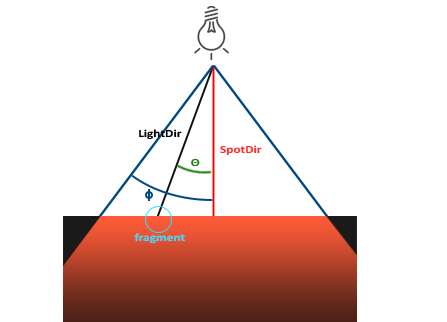1. $\vec{LightDir}$从Fragment指向光源的向量
2. $\vec{SpotDir}$是聚光照亮的方向
3. $\phi$是切光角，用来制定聚光的半径，半径之外是漆黑的。
4. $\theta$是$\vec{LightDir}$与$\vec{SpotDir}$的夹角，如果在聚光灯之内其值应该小于切光角

### 手电筒struct Light {
vec3 position;
vec3 direction;
float cutOff;

vec3 ambient;
vec3 diffuse;
vec3 specular;
};

...
float theta = dot(lightDir, normalize(-light.direction));
if(theta > light.cutOff) {
// do lighting calculations
} else {
// use ambient light so scene isn't completely dark outside the spotlight.
color = vec4(light.ambient * vec3(texture(material.diffuse, TexCoords)), 1.0);
}


glUniform3f(glGetUniformLocation(shaderProgram, "light.position"), cameraPos.x, cameraPos.y, cameraPos.z);### 模糊边缘

内圆锥我们用上面的就行，但还需要一个光线渐弱的外圆锥

$I = \frac{\Theta - \gamma}{\epsilon}$

1. $\theta$是$\vec{LightDir}$与$\vec{SpotDir}$的夹角, 即照亮Fragment的光线与光源照射方向的夹角
2. $\gamma$(gamma)外圆锥的$\cos$值
3. $\epsilon$(epsilon)是内圆锥$\phi$(phi)与外圆锥$\gamma$(gamma)的$\cos$差值
$\theta$ $\theta$(度) $\phi$(内圆锥) $\phi$(度) $\gamma$(外圆锥) $\gamma$(度) $\epsilon$ I
0.87 30 0.91 25 0.82 35 0.91 - 0.82 = 0.09 0.87 - 0.82 / 0.09 = 0.56
0.9 26 0.91 25 0.82 35 0.91 - 0.82 = 0.09 0.9 - 0.82 / 0.09 = 0.89
0.97 14 0.91 25 0.82 35 0.91 - 0.82 = 0.09 0.97 - 0.82 / 0.09 = 1.67
0.83 34 0.91 25 0.82 35 0.91 - 0.82 = 0.09 0.83 - 0.82 / 0.09 = 0.11
0.64 50 0.91 25 0.82 35 0.91 - 0.82 = 0.09 0.64 - 0.82 / 0.09 = -2.0
0.966 15 0.9978 12.5 0.953 17.5 0.9978 - 0.953 = 0.0448 0.966 - 0.953 / 0.0448 = 0.29

float theta     = dot(lightDir, normalize(-light.direction));
float epsilon   = light.cutOff - light.outerCutOff;
float intensity = clamp((theta - light.outerCutOff) / epsilon, 0.0, 1.0);
...
// we'll leave ambient unaffected so we always have a little light.
diffuse  *= intensity;
specular *= intensity;
...


glUniform1f(glGetUniformLocation(shaderProgram, "light.outerCutOff"), glm::cos(glm::radians(17.5f)));